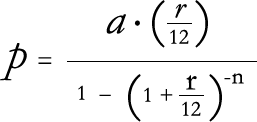# Loan Calculator

Instructions

This loan calculator can be used to calculate an unknown variable in a loan scenario where an amount of money (loan amount) is loaned to a borrower who is charged an interest rate that is compounded monthly while repaying the loan with monthly payments until the principle balance of the loan amount is zero. To use the loan calculator:

1. Make sure the field for the variable you wish to calculate is blank. If it is not, delete all characters from that field.
2. Enter known values into the remaining three fields.
NOTE: The interest rate should be entered as a percentage, and you must select either "NOM" for nominal annual interest rate or "APR" for annual percentage rate. (See below for definitions.) For example, if your bank charges a nominal annual interest rate of 6.35%, enter 6.35 in the interest rate field, not 0.0635 like some calculators require, and select "NOM" in the drop-down menu.
3. Click the "Calculate Unknown Variable" button.

NOTE: Where APR is used, this calculator does not include non-interest components of APR (annual percentage rate), like origination fees, points, insurance, and other fees.

Definitions

Loan Amount: This is the amount of the loan, also known as present value or initial principal balance.

Months: The number of months in the term (length) of the loan. Since payments are assumed to be monthly, the number of months is also the number of payments.

Payment: The amount that must be repaid each month to cover interest that has accrued for that month and also repay some of the principal balance so that the loan is completely repaid by the end of the total months. The payment size will be the same each month, except the final payment may be a partial payment.

Interest Rate: The percentage of the principal balance that is charged each month expressed as an annual rate. The interest rate is the primary way that a lender charges a borrower. This calculator allows both nominal annual interest rate (NOM) and annual percentage rate (APR) to be used for interest rate in calculations.

Nominal Annual Interest Rate (NOM): Stated interest rate without adjustment for the full effect of compounding schemes. An interest rate is considered a nominal rate if the frequency of compounding (e.g. each month) is not identical to the basic time unit (one year in the case of annual interest rates). This calculator assumes that, when nominal annual interest rate is used, the payment and the compounding of interest coincide at the same frequency—monthly. The nominal annual interest rate is divided by 12 to determine the percentage of the principal balance that is charged as interest for a particular payment.

Annual Percentage Rate (APR): The effective annual interest rate after taking into account the compounding scheme used by the lender. Also referred to as "real" interest rate or "actual" interest rate. APR allows borrowers to compare rates from different institutions without having to consider the compounding scheme. In other words, APR allows borrowers to compare apples with apples, so to speak.

Tips for Mobile Device Users

If you would like to use this loan calculator on your mobile phone or other mobile device, just open this web page in your mobile device's web browser. Then bookmark the page or use your favorite method to add an icon to your mobile device's home screen/menu.

What makes this calculator different than the rest?

• It's formatted nicely for mobile devices, especially mobile phones like Android and iPhone.
• It does not require JavaScript, Flash, Java, or any other web browser add-ons.
• It works in 100% of all web browsers.
• It's 100% valid HTML 5 and CSS according to W3C standards.
• It's simple, fast, and easy to use.
• It allows the user to input either the nominal annual interest rate (NOM) or annual percentage rate (APR) when solving for loan amount, payment, or months.
• When solving for interest rate, both nominal annual interest rate (NOM) and annual percentage rate (APR) are calculated.
• It uses "Vieth's Practical Present Value of an Annuity Formula for Loans With Monthly Payments." Vieth's Formula is presented below. ('p' represents monthly payment, 'a' represents the amount of the loan, 'r' represents nominal annual interest rate (NOM) as a decimal number, e.g. 0.0635 for 6.35%, and 'n' represents number of monthly payments.)Please Support This Application!

This is a FREE web application. If you find it useful, please consider donating funds to support web hosting for this app.PHP Source Code Available

The PHP source code for this loan calculator is available for use in other applications or as a code example. A competent developer can very easily convert the PHP source code to Java, JavaScript, C#, or any other modern programming language. Terms of use vary depending on the application. For more information about obtaining the PHP source code for this web application, send e-mail to John@MasterWebsmith.com.

Contact Information

Questions? Comments? Send e-mail to John@MasterWebsmith.com.

Copyright © 2021 John A. Vieth
Web hosting by Master Websmith